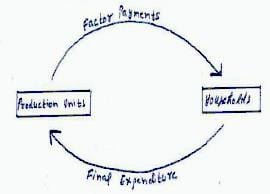# Scanner - Introduction and Circular Flow of Income (2016-2018) Notes | Study Crash Course of Macro Economics -Class 12 - Commerce

## Commerce: Scanner - Introduction and Circular Flow of Income (2016-2018) Notes | Study Crash Course of Macro Economics -Class 12 - Commerce

The document Scanner - Introduction and Circular Flow of Income (2016-2018) Notes | Study Crash Course of Macro Economics -Class 12 - Commerce is a part of the Commerce Course Crash Course of Macro Economics -Class 12.
All you need of Commerce at this link: Commerce

SAMPLE PAPER’S & C.B.S.E 2016

(Q1)  Define flows.       (1M)

Ans: Flows are variables whose magnitude is measured over a period of  time

(Q2) Define Stock

Ans: Stocks are variable whose magnitude is measured at a point of time

(Q3) Define nominal flow.   (1M)

Ans: Nominal Flow/Money Flow is the flow of factor payments and payments for goods and services between households & firms.

(Q4) Explain the circular flow of income.

Ans: Production units produce goods and services by employing factor of production from households. These units in turn make factor payments to households. Households spend this income on goods and services produced by households. This makes the circular flow complete.

Incomes are first generated in production units due to the joint efforts of factor owners from households. These incomes are distributed to the factor owners who in turn spend the income on purchasing goods and services produced in production units. This makes the circular flow of income complete. (Explanation without the use of diagram must be awarded)(Q5)   Which of the following is a stock ? (Choose the correct alternative)

(a) Wealth

(b) Saving

(c) Exports

(d) Profits

(Q6) Which of the following is not a flow ?

(a) Capital

(b) Income

(c) Investment

(d) Depreciation

Ans: 5 (a)  , 6 (a)

CBSE 2017

(Q1) Distinguish between stocks and flows. Give an example of each.            (3M)

Ans: Stocks are variables measured at a point of time, whereas, flows are variables               measured over a period of time.  (2M)

Example     Stock - Population, etc

Flow - Growth rate of Population, etc .(1/2x2M)

(Q2) Explain the circular flow of income.

(Q3) There are ……… phases in the circular flow of income.

(a) 2

(b) 3

(c) 4

(d) 5

(Q4) Distribution phase of circular flow of income involves

(a) Production of goods and services

(b) Flow of factor income

(c) Expenditure on goods and service

(d) None of these

(Q5) Circular flow of income

(a) Gives information about injections and leakages from the flow of income.

(b) Enables estimation of national income.

(c) Provides knowledge of interdependence among different sectors.

(d) All of these

(Q6) From the macro point of view, an economy is divided into …. Sectors.

(a) 2

(b) 3

(c) 4

(d) 5

(Q7) Continuous sectoral flow of goods and money I an economy is known as _____

(a) Real flo

(b) Nominal flow

(c) Circular flow

(d) None of these

(Q8) Continuous sectoral flow of goods and services in an economy is know as ____

(a) Real flow

(b) Nominal flow

(c) Circular flow

(d) None of these

(Q9) In a two-sector economy, the condition of equilibrium is :

(a) I = S

(b) I + G = S + T

(c) I + G + X = S + T + M

(d) C+ I = I + S

Ans: 3 (b) , 4 (b) , 5 (d) , 6(c) , 7 (c) , 8(a), 9 (a)

(Q10) Real flow is also known as nominal flow.

Ans: False.

(Q11) Generation and distribution are the two phases in circular flow of income.

Ans: False.  There are 3 different phases (generation, distribution and disposition) in circular flow of income..

Previous C.B.S.E & N.C.E.R.T QUESTION ’S

(Q1) Define Macro economics ?

(Q2) Give two examples Of Macro economics and two example of micro economics ?

(Q3) State related branch of economics for

(a) Cotton Textile Industry

(b) Price determination of a commodity

(Q4) Why is study of the problem of unemployment in India considered a macro economic study

(Q5) Give two examples of the study of aggregates of the economic system ?

(Q6) Is Study of problems of agricultural labour in India a macroeconomics study ?

(Q7) Is Study of wages of Computer Engineer  in India a microeconomics study ?

(Q8) State whether the following are Stock or Flow Variables

(a) Population

(b)  Wealth

(c) No. of Birth

(d) Cement Production

(e) Investment

(f) Expenditure on food by household

(g) Deposit in saving account of a Bank

N.C.E.R.T QUESTION ’S

(Q1) What is the principle of circular flow of income and product ?

(Q2) Describe the four major sectors in an economy according to the macro economic point of view

(Q3) Why should the aggregate final expenditure of an economy be equal to the Aggregate factor payment ?

(Q4) Distinguish between stock and flow ? Between net investment and capital which is stock and which is flow ? Compare it with flow of water into the tank ?

The document Scanner - Introduction and Circular Flow of Income (2016-2018) Notes | Study Crash Course of Macro Economics -Class 12 - Commerce is a part of the Commerce Course Crash Course of Macro Economics -Class 12.
All you need of Commerce at this link: CommerceUse Code STAYHOME200 and get INR 200 additional OFF

## Crash Course of Macro Economics -Class 12

34 docs|4 tests

Track your progress, build streaks, highlight & save important lessons and more!

,

,

,

,

,

,

,

,

,

,

,

,

,

,

,

,

,

,

,

,

,

;